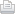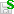Journal cover Journal topic
Hydrology and Earth System Sciences An interactive open-access journal of the European Geosciences Union
Journal topic

Journal metrics

•IF 4.936
•IF 5-year
5.615
•CiteScore
4.94
•SNIP 1.612
•IPP 4.70
•SJR 2.134
•Scimago H
index 107
•h5-index 63

Abstracted/indexed

Abstracted/indexed
https://doi.org/10.5194/hess-2019-283https://doi.org/10.5194/hess-2019-283Submitted as: research article 09 Jul 2019

Submitted as: research article | 09 Jul 2019

Review status
This discussion paper is a preprint. It is a manuscript under review for the journal Hydrology and Earth System Sciences (HESS).

Towards understanding the mean annual water-energy balance equation based on an Ohms-type approach

Xu Shan, Xingdong Li, and Hanbo Yang Xu Shan et al.
• State Key Laboratory of Hydro-Science and Engineering, Department of Hydraulic Engineering, Tsinghua University, Beijing 100084, China

Abstract. The Budyko hypothesis has been widely used to describe precipitation partitioning at the catchment scale. Many empirical and analytical formulas have been proposed to describe the Budyko hypothesis. Based on dimensional analysis and mathematic reasoning, previous studies gave an analytical derivation, i.e., the Mezentsev-Choudhury-Yang (MCY) equation. However, few hydrological processes were involved in the derivation. Therefore, this study firstly defines a catchment network to describe water vapor transformation and transportation using the Lagrangian particle tracking method; and then proposes the generalized flux of water vapor, which can be expressed as the ratio of potential difference with resistance. Furthermore, this study obtains a new constraint for the mean annual water-energy balance, 1 f(E)  =  1 f(E0)  +  1 f(P) with E, E0 and P being evaporation, potential evaporation and precipitation, respectively, and f( ) being a function of generalized flux, based on an analogy of the Ohms-type approach and the homogeneity assumption, i.e., the generalized flux has the same form for both water vapor transportation and chase transformation, and in other words, precipitation and potential evaporation have an equalized effect on evaporation. According to this constraint, the MCY equation can be obtained when the generalized flux f( ) is a power function. In addition, this study suggests a more general expression E  =  P(b+kE0) [pn+(b+kE0n]1/n under conditions without the homogeneity constraint, where E, E0 and P are evaporation, potential evaporation and precipitation, respectively, and n, k and b are constants (MCY equation when b = 0 and k = 1).

Xu Shan et al.
Interactive discussion
Status: open (until 08 Nov 2019)
Status: open (until 08 Nov 2019)
AC: Author comment | RC: Referee comment | SC: Short comment | EC: Editor comment- Printer-friendly version- Supplement
Xu Shan et al.
Xu Shan et al.
Viewed
Total article views: 450 (including HTML, PDF, and XML)
HTML PDF XML Total BibTeX EndNote
376 68 6 450 3 6
• HTML: 376
• PDF: 68
• XML: 6
• Total: 450
• BibTeX: 3
• EndNote: 6
Views and downloads (calculated since 09 Jul 2019)
Cumulative views and downloads (calculated since 09 Jul 2019)
Viewed (geographical distribution)
Total article views: 312 (including HTML, PDF, and XML) Thereof 305 with geography defined and 7 with unknown origin.
Country # Views %
• 1
1

Cited
Saved
No saved metrics found.
Discussed
No discussed metrics found.
Latest update: 13 Oct 2019
Short summary
The Budyko hypothesis has been generally used to quantify how much precipitation transforms into evaporation in one catchment. To approach this hypothesis, previous studies proposed analytical formulas derived based on mathematic reasoning. Differently, this study drew a new derivation for this hypothesis based on fundamental physical principles. It clearly reveals the underlying assumptions in the previous mathematic reasoning and promotes hydrologic understanding on this hypothesis.
The Budyko hypothesis has been generally used to quantify how much precipitation transforms into...
Citation